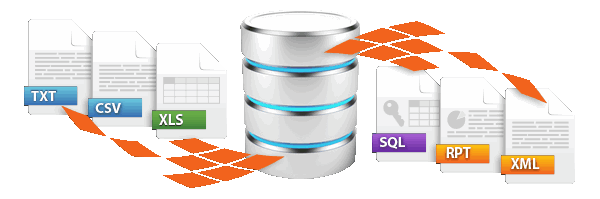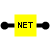Dynamic data import / exportsimply more productive
Up to 100,000 records can be processed with the freeware version!

# .NET Script Heater, dynamic .NET Script CodeThe .NET Script Heater enables you to equip your FlowHeater Definition with any desired .NET code. This means that there is no limit to the kinds of dynamic transformation possible.

Anything that cannot be achieved using the (extensive) functions (Heaters) that come with FlowHeater, you can program for yourself with the help of the .NET Script Heater straightforwardly in DOT.NET. Both the programming languages of C# (C-sharp) and VB.NET (Visual Basic) are supported.

Note: Some extremely complex scripts can be used. Even complete classes can be included as source.

The .NET Script Heater properties popup is really easy to operate. Using the Check Syntax button the code is compiled and, if applicable, errors are reported.

The .NET Script Heater makes use of the same mechanisms as FlowHeater itself. Incoming data types are automatically converted into the necessary data types according to the format settings on the WRITE Adapter. In order to make use of these, five functions are available.

Function
Description
object InValues[x].GetValue()

Returns the raw contents of the parameter without conversion, you have to handle these yourself.

object InValues[x].GetInt()

Converts the contents to an Integer value.

object InValues[x].GetLong()

Converts the contents to an Long value.

object InValues[x].GetBool()

Converts the contents to a Boolean value.

object InValues[x].GetDouble()

Converts the contents to a Double value.

object InValues[x].GetDecimal()

Converts the contents to a Decimal value.

object InValues[x].GetString()

Converts the contents into a String.

object InValues[x].GetDateTime()

Converts the contents into a DateTime type.

Note: x = 0 is the first parameter

Warning: In all functions, if the return value is NULL then the contents could not be converted.

The heater parameters can be accessed using the InValues array with the methods listed above. The sequence of parameters is the same as defined in Designer.

#### Return value

The result of your own transformation function is delivered as a return value for further processing by FlowHeater. The following .NET data types can be returned at present.

• null
• bool
• int
• long
• double
• decimal
• DateTime
• string

#### Examples

##### or inspect the following short C# (C-sharp) Script code extracts for a brief introduction

Simple counter, returning 1, 2, 3, and so on… according to the number of records processed.

int i = 0;
public object DoWork()
{
return ++i;
}

Extended counter including invocation of an additional function. Returning this time 2, 4, 6, and so on... according to the number of records processed.

int i = 0;
public object DoWork()
{
return Calculate();
}

private int Calculate ()
{
i += 2;
return i;
}

A somewhat more complex calculation using an additional class, which processes the first heater parameter.

public object DoWork()
{
object o = InValues.GetInt();
if (o == null)
return -1;
else
{
ComplexCalculation complex = new ComplexCalculation();
return complex.Calculate((int)o);
}
}

public class ComplexCalculation
{
public ComplexCalculation()
{
}

public int Calculate (int i)
{
return i + i;
}
}

### Imported namespaces and references

At present a fixed reference to the .NET System.dll is used, with this the following Namespaces

System
System.Text
System.IO
System.Reflection
System.Text.RegularExpressions

are imported. In a later version, it is planned to make it possible to configure references and imported namespaces in the properties popup window. This will also make it possible to integrate references to you own .NET DLLs.

Please also refer to the general information on the use of Heaters (functions)